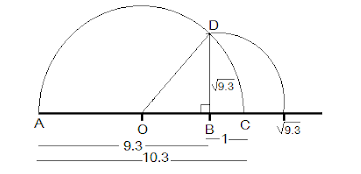Newbie

# Represent (√9.3) on the number line. Q.4

• 0

Find the question of exercise 1.5 question 4 of chapter 1.5 of ncert class 9th . Sir please help me to solve the important question its very tough to solve please give me the best solution of this question also find the simplest method of  this question.Represent (√9.3) on the number line

Share

1. Solution:

Step 1: Draw a 9.3 units long line segment, AB. Extend AB to C such that BC=1 unit.

Step 2: Now, AC = 10.3 units. Let the centre of AC be O.

Step 3: Draw a semi-circle of radius OC with centre O.

Step 4: Draw a BD perpendicular to AC at point B intersecting the semicircle at D. Join OD.

Step 5: OBD, obtained, is a right angled triangle.

Here, OD 10.3/2 (radius of semi-circle), OC = 10.3/2 , BC = 1

OB = OC – BC

⟹ (10.3/2)-1 = 8.3/2

Using Pythagoras theorem,

We get,

OD2=BD2+OB2

⟹ (10.3/2)2 = BD2+(8.3/2)2

⟹ BD2 = (10.3/2)2-(8.3/2)2

⟹ (BD)= (10.3/2)-(8.3/2)(10.3/2)+(8.3/2)

⟹ BD2 = 9.3

⟹ BD =  √9.3

Thus, the length of BD is √9.3.

Step 6: Taking BD as radius and B as centre draw an arc which touches the line segment. The point where it touches the line segment is at a distance of √9.3 from O as shown in the figure.• 0